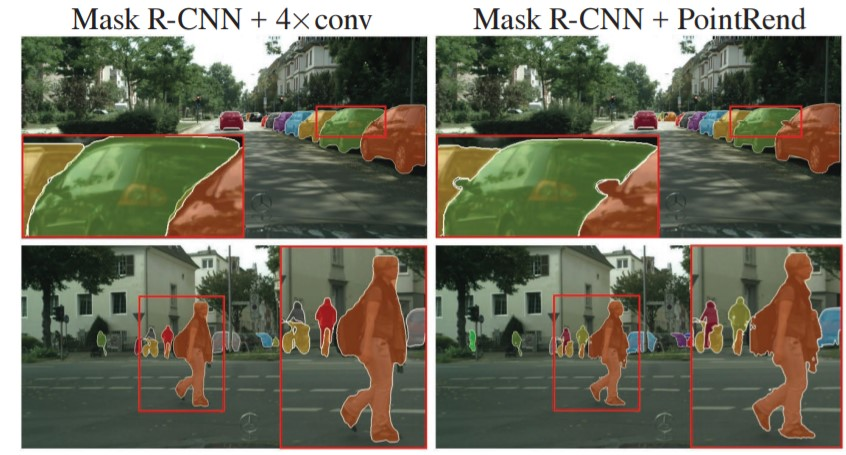### ArcGIS API for Python

• Deep Learning with ArcGIS
• 2D Computer Vision
• Object Detection

### Instance segmentation

In our guide titled How SSD Works, we learned how SSD detects objects and also finds their position in terms of bounding boxes. This class of algorithm is called Object Detection.

In another guide titled How U-net Works, we saw how to achieve pixel level classification that helps in solving problems like land cover classification. Algorithms achieving tasks like this are categorized as Semantic Segmentation.

Object Instance Segmentation is a recent approach that gives us best of both worlds. It integrates object detection task where the goal is to detect object class along with bounding box prediction in an image and semantic segmentation task, which classifies each pixel into pre-defined categories Thus, it enables us to detect objects in an image while precisely segmenting a mask for each object instance.

Instance segmentation allows us to solve problems like damage detection where it's important to know extent of damage. Another use case is in case of self driving cars where it's important to know position of each car in the scene. Generating building footprints for each individual building is a popular problem in the field of GIS. `arcgis.learn` gives us advantage to use Mask R-CNN model to solve such real life problems.

Let us take an example of building footprint detection use case.Figure 1:

Segmentation Types

Image (a) has two type of pixels. One belongs to the object (Building) and other belongs to background. It is difficult to count the number of buildings present in the image. In image (b) each building is identified as distinct entity hence overcomes the limitation of semantic segmentation.

Mask R-CNN is a state of the art model for instance segmentation, developed on top of Faster R-CNN. Faster R-CNN is a region-based convolutional neural networks , that returns bounding boxes for each object and its class label with a confidence score.

To understand Mask R-CNN, let's first discus architecture of Faster R-CNN that works in two stages:

Stage1: The first stage consists of two networks, backbone (ResNet, VGG, Inception, etc..) and region proposal network. These networks run once per image to give a set of region proposals. Region proposals are regions in the feature map which contain the object.

Stage2: In the second stage, the network predicts bounding boxes and object class for each of the proposed region obtained in stage1. Each proposed region can be of different size whereas fully connected layers in the networks always require fixed size vector to make predictions. Size of these proposed regions is fixed by using either RoI pool (which is very similar to MaxPooling) or RoIAlign method.Figure 2:

Faster R-CNN is a single, unified network for object detection 

Faster R-CNN predicts object class and bounding boxes. Mask R-CNN is an extension of Faster R-CNN with additional branch for predicting segmentation masks on each Region of Interest (RoI).Figure 3:

The Mask R-CNN framework for instance segmentation 

In the second stage of Faster R-CNN, RoI pool is replaced by RoIAlign which helps to preserve spatial information which gets misaligned in case of RoI pool. RoIAlign uses binary interpolation to create a feature map that is of fixed size for e.g. 7 x 7.

The output from RoIAlign layer is then fed into Mask head, which consists of two convolution layers. It generates mask for each RoI, thus segmenting an image in pixel-to-pixel manner.

### PointRend Enhancement

Segmentation models can tend to generate over-smooth boundaries which might not be precise for objects or scenes with irregular boundaries. To get a crisp segmentation boundary, a point-based rendering neural network module called PointRend has been added as an enhancement to the existing model. This module draws methodology from classical computer graphics and gives the perspective of rendering to a segmentation problem. Image segmentation models often predict labels on a low-resolution regular grid, for example, 1/8th of the input. These models use interpolation to upscale the predictions to original resolution. In contrast, PointRend uses iterative subdivision algorithm to upscale the predictions by predicting labels of points at selected locations by a trained small neural network. This method enables high-resolution output in an efficient way.Figure 4:

PointRend enhancement (right) over original segmentation model (left) 

To enable PointRend with MaskRCNN, initialize the model with parameter `pointrend=True`:

``````model = MaskRCNN(data=data, pointrend=True)
``````

 Kaiming He, Georgia Gkioxari, Piotr Dollár, Ross Girshick: “Mask R-CNN”, 2017; [http://arxiv.org/abs/1703.06870 arXiv:1703.06870].

 Shaoqing Ren, Kaiming He, Ross Girshick, Jian Sun: “Faster R-CNN: Towards Real-Time Object Detection with Region Proposal Networks”, 2015; [http://arxiv.org/abs/1506.01497 arXiv:1506.01497].

 Alexander Kirillov, Yuxin Wu, Kaiming He, Ross Girshick: “PointRend: Image Segmentation as Rendering”, 2019; [http://arxiv.org/abs/1912.08193 arXiv:1912.08193].